# Bihar Board 12th Chemistry Important Questions Long Answer Type Part 5 in English

BSEB Bihar Board 12th Chemistry Important Questions Long Answer Type Part 5 are the best resource for students which helps in revision.

## Bihar Board 12th Chemistry Important Questions Long Answer Type Part 5 in English

Question 1.
Describe the applications of Kholramch’s law.
Following are the applications of Kohlrausch’s law :
(a) In Calculating the molar conductivity of a weak electrolyte at infinite dilution.
Kohlrausch’s law is helpful in determining the limiting molar conductivities if weak electrolytes. The molar conductivity at infinite delution (Aµ) form weak electrolytes cannot be obtained directly by the extrapolation of plot of Am versus $$\sqrt{C}$$, But this can be easily calculated with the help of kohlrausch’s law.

For example, the value of Am for acetic acid (CH3COOH) can be calculated form the knowledge of the molar conductivities at infinite dilution of strong electrolytes like CH3COONa, HCl and NaCl as follows: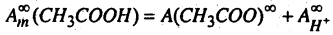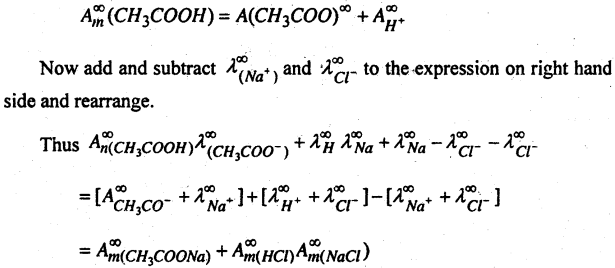(b) Calculation of degree of dissociation of weak electrolyte- Molar conductivity of an electrolyte depends upon its degree of dissociation. Higher the degree of dissociation, higher is the molar conductivity. As dilution increases, the degree of dissociation of weak electrolyte also increases and consequently, molar conductivity of electrolyte increases. At infinite dilution molar conductivity becomes maximum because degree of dissociation approaches unity.

Thus if Acm = molar conductivity of solution at any concentration
Ac = molar conductivity at infinite dilution
α = degree of dissociation
α = $$\frac{A_{m}^{c}}{A_{m}^{\infty}}$$

Question 2.
Physical adsorption- When particles of the adsorbate and held to the surface of the adsorbent by the weak forces such as Vande Waals force the adsorption is called physical adsorption of physisorption.

Chemical adsorption- When the molecular of the adsorbate are held to the surface of adsorbent by the chemical forces, the adsorption is called chemical adsorption. In case a chemical reaction occurs between the adsorbed molecules and the molecules or atoms of adsorbent. It is also known as chemisorption.

Difference between physical and chemical adsorption

1. Enthalpy of adsorption usually is the order of 20-40 kJ mol-1
2. Moles of adsorbate and adsorbent are held by Van der Waals interactions.
3. It usually takes place at low temperature and decreases with increasing temperature.
4. It is not very specific i.e. all gases are adsorbed at all solids to some extent.
5. Multi-molecular layers may be formed on the adsorbent.
6. It does not require any activation energy
7. The amount of adsorbed in related to the case of liquefaction of the gas.
8. It is reversibre in nature.

1. Enthalpy of adsorption is of the order 40-400 kJ mol-1
3. It takes place are relatively high temperatures.
4. It is highly specific and takes place when there is some possibility of compound formation between the adsorbent molecules
5. Usually mono-molecular layer is found on the adsorbent.
6. It requires activation energy
7. There is no such correlation.
8. It is irreversible in nature.

Question 3.
(A) How can you prepare alcohols from (1) alkyl halide (2) Aldehydes and ketones.
(B) Discuss the following chemical reaction of alcohols (1) Oxidation (2) Reaction with PCl5 (3) Esterification and (4) Dehydration.
(A) Methods of Preparation of alcohols-
(1) From Alkyl halides Haloalkancs-
When alkyl halide is boiled with aqueous solution of an alkali hydroxide or moist silver oxide give primary alcohols.
RX + KOH(aq.) → ROH + KX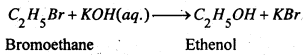(2) From Aldehydes and ketones-
(a) Using Grignard’s reagent- Grignard reagents react with aldehydes and ketones to form products which decomposes with dil HCl or dil H2SO4 to give primary, secondary and tertiary alcohols.
Formaldehyde gives primary alcohol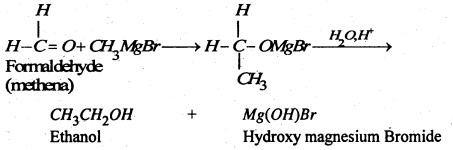(b) By reduction- Aldehydes on reduction give primary alcohols and ketones furnish secondary alcohols.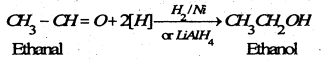(B) Chemical reaction of alcohols-
(1) Oxidation- (a) A primary alcohol on Oxidation with acidified potassium dichromate first gives an aldehyde which on further Oxidation gives an acid with the same number of carbon atom as the original alcohol.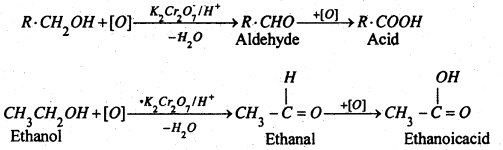(2) Reaction with PCl5- Alcohols react with PCl5 to form chloroalkane :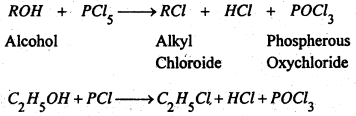(3) Esterification- Alcohols react with monocarboxylic acids in the presence of conc. H2SO4 to form ester. This reaction is called “esterification.”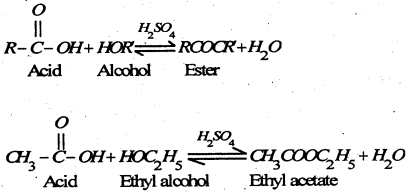(4) Dehydration- When alcohol is heated with cone. H2O4, Phosphoric acid or boric acid, it undergo dehydration to form alkenes. The reaction with cone. H2SO4 is carried at 443K, whereas phosphoric acid and boric acid react at higher temperature.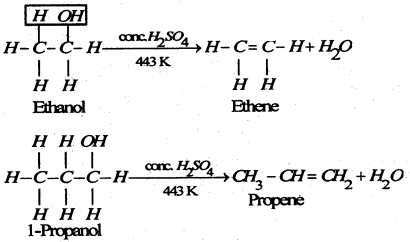The case of dehydration of alcohols follows the order:
Tertiary alcohol > Sec. alcohol > prim-alcohol.

Question 4.
Write Principle Involved in the manufacture of Nitric acid (HNO3) by Ostwaid Process.
In modem time, Nitric acid is manufactured by Ostwald process in large extent & Nitric acid is manufactured in following steps:
Step-I: The mixture of Ammonia acid air passed over platinum gauze catalyst at 750 – 900°C Ammonia is oxidised to Nitric Oxide.The reaction is exothermic, the heat of reaction maintains the temperature of the catalyst.

Step-II: The Nitric Oxide is then oxidised to NO2 by air which is cooled to 50°C and absorbed in water.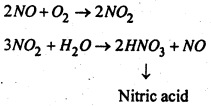Question 5.
(A) What do you mean by lowering of vapour pressure and relative lowering of vapour pressure?
(B) Describe the Raoult’s law of relative lowering of vapour pressure. How is it related to the molecular weight of solute?
(A) Lowering of vapour pressure- First of all Gay-Lussac found that, when a non-volatile solute is dissolved in a liquid (solvent) then at same temperature and pressure the vapour pressure of solution became lower than the vapour pressure of pure solvent. The lowering in vapour pressure of a solution is directly proportional to the amount of solute. Thus “the difference of vapour pressure of solvent and vapour pressure of solution at a given temperature is called lowering of vapour pressure”.

If the vapour pressure of a solvent = po and the vapour pressure of solution V = Ps at given temperature. Then lowering of vapour pressure = (po = ps).

Relative lowering of vapour pressure- The vapour pressure of a liquid (solvent) is lowered when a non-volatile solute is dissolved in it and the lowering of the vapour pressure is proportional to the weight of the solute dissolved. Suppose the pure liquid has a vapour pressure p0 and the solution of a known concentration at the same temperature has a vapour pressure ps The lowering of vapour jpressure is po – ps. It was shown by Von Babo (1848) that although the value of pa increases rapidly with the temperature but the ratio $$\frac{p_{o}-p_{s}}{p_{o}}$$ remains the same at all temperatures for a given solution. This ratio is termed as die relative lowering of the vapour pressure. Thus, “the ratio of the lowering of the vapour pressure to the vapour pressure of the pure solvent at the same temperature is known as the relative lowering of the vapour pressure.” i.e. $$\frac{p_{o}-p_{s}}{p_{o}}$$

(B) Raoult’s Law- Raoult carried extensive researches on the vapour pressures of dilute solutions and in 1887 established the fundamental law governing the vapour pressure of dilute solutions. This is known as Raoult’s Law and maybe states as-

“The relative lowering of vapour pressure is equal to the molar fraction of the solute”.
Molar fraction is defined as the ratio of the number of molecules of the solute to the total number of molecules in the solution i.e. if V is the number of solute molecules dissolved in ‘If molecules of the solvent, then the molar fraction of the solute is-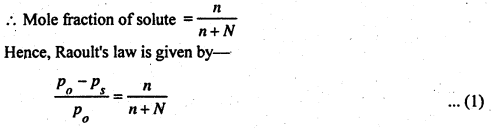Relation between relative low ering of Vapour pressure and Molecular weight of solute-
We have from Raoult’s law-
$$\frac{p_{o}-p_{s}}{p_{o}}=\frac{n}{n+N}$$
If solution contains, solute and Wg solvent. The molecular weight of solute is ‘m‘ and the molecular weight of solvent is M,
then n = $$\frac{w}{m}$$ and N = $$\frac{W}{M}$$
Substituting the value of n and N in the above equation, we have-
$$\frac{p_{o}-p_{s}}{p_{0}}=\frac{w / m}{w / m+W / M}$$

But in case of very dilute solution, V may be neglected (because it is very small) from the denominator. Thus Raoult’s law can be written as: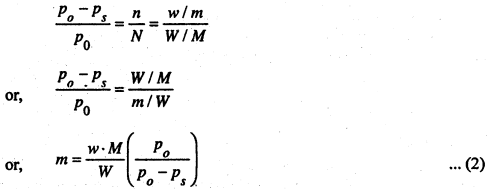If the value of w, W, M, po be known, we can calculate the molecular weight of solute from eqn. (2).

Question 6.
(A) Define the following :
(1) Conductivity (2) Specific conductivity (3) Equivalent conductivity and (4) Molecular conductivity.
(B) What do you mean by electrode potential?
(A) (1) Conductivity: Reciprocal of resistance is called conductivity of the conductor.
Conductivity (C) = $$\frac{1}{\text { Resistance }(R)}$$

(2) Specific Conductivity:
Like a metallic conductor, the resistance R offered by an electrolyte to the passage of electric current is proportional to the length L and inversely proportional to the area of cross section a, of the column of the electrolyte lying between the electrodes i.e.,
R = $$\frac{1}{a}$$
or, R = ρ × $$\frac{l}{a}$$ ……….(i)

where ρ is const. called specific resistance.
“The reciprocal of specific resistance is known as specific conductivity”
Substituting the terms conductivity and specific conductivity in (i) we get-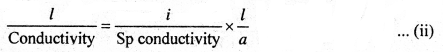when l = 1 cm., a = 1 sq. cm., then (ii)
becomes-
Sp. Conductivity = Conductivity
Hence specific conductivity may be defined as:
“The conductivity of 1 cm. cube of the solution of electrolyte”.
It is denoted by symbol kv when V is the volume in cc., containing a certain weight of
substance dissolved in it and is measured in reciprocal ohms or mhos.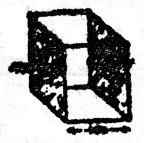(3) Equivalant conductivity : To compare the current conducting capacities of electrolytes Kohlrausch adopted a function called equaivalent conductivity and may be defined as : –

“The conductivity of solution containing 1 cm. equivalent of an electrolyte when placed between two sufficient large electrodes which are 1 cm. apart is known as equivalent conductivity of the solution”.

It is denoted by where λ1 is the volume in the containing 1 g. equivalent dissolved in it and is measured in mhos.
Since the conductivity of V. c. c. solution between electrodes 1 cm. apart may be taken as V times the conductivity of 1 c.c. solution (i.e. specific conductivity), hence λ1 is equal to V times the specific conductivity,
or, lv =Kv × V

Thus the equivalent conductivity of a solution may also be defined as:
“The product of specific conductivity and the volume V in c.c. of the solution containing g. equivalent of the electrolyte”.

(4) Molecular conductivity it may be defined as :
“The conductivity of a solution containing 1 gm. mol. of the electrolyte when placed between two sufficiently large electrodes one cm. apart is known as molecular conductivity of the solution”.
It is denoted by symbol μv and is measured in mhos. It is numerically equal to :
“The product of specific conductivity and the volume V in c.c. of the solution containing 1 g. mol. of the electrolyte”.
or, µv = Kp × V

(C) Electrode potential: When a metal electrode is placed in a solution of an electrolyte having ions of the same metal, an electrical potential difference is developed at the separating layer of metal, electrode and solution. This electrical potential difference is called the electrode potential.

It is obvious that each cell is made up of two metal electrodes. At one electrode oxidation takes place (i.e. electrons are used up).

For example: Daniell Cell, in which oxidation takes place at zinc electrode while reduction at copper electrode is known as half cell. The potential between a metal and a solution is known as single electrode potential.

In this way each electrode of the cell exerts an electrode potential at the junction of metal and solution. Thus the e. m. f. the cell may be regarded as the algebraic sum of two electrode potentials i.e. the single electrode potential of cathode and anode respectively. Hence-
$$E_{\text {cell }}=E_{\text {anode }}+E_{\text {cathode }}$$

It is not possible to determine experimentally, the single electrode potential (i.e. of a half cell). Usually a half cell is combined with some standard electrode and the e.m.f. of the complete cell is measured.